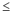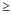# Manually calculate a p-value

## Introduction to calculating a p-value

The p-value is calculated using the sampling distribution of the test statistic under the null hypothesis, the sample data, and the type of test being done (lower-tailed test, upper-tailed test, or two-sided test).

The p-value for:
• a lower-tailed test is specified by: p-value = P(TSts | H0 is true) = cdf(ts)
• an upper-tailed test is specified by: p-value = P(TSts | H0 is true) = 1 - cdf(ts)
• assuming that the distribution of the test statistic under H0 is symmetric about 0, a two-sided test is specified by: p-value = 2 * P(TS|ts| | H0 is true) = 2 * (1 - cdf(|ts|))
Where:
P
Probability of an event
TS
Test statistic
ts
observed value of the test statistic calculated from your sample
cdf()
Cumulative distribution function of the distribution of the test statistic (TS) under the null hypothesis

Minitab automatically displays p-values for most hypothesis tests. But you can also use Minitab to “manually” calculate p-values. To manually calculate a p-value in Minitab:

1. Choose Calc > Probability Distributions > Choose the appropriate distribution.
2. Choose Cumulative probability.
3. Provide the parameters if necessary.
4. Choose Input constant and enter the test statistic.
5. Click OK.
The result (cdf(ts)) is the probability that the test statistic is equal to or less than that value actually observed based on your sample under H0.
• For a lower-tailed test, the p-value is equal to this probability; p-value = cdf(ts).
• For an upper-tailed test, the p-value is equal to one minus this probability; p-value = 1 - cdf(ts).
• For a two-sided test, the p-value is equal to two times the p-value for the lower-tailed p-value if the value of the test statistic from your sample is negative. However, the p-value is equal to two times the p-value for the upper-tailed p-value if the value of the test statistic from your sample is positive.

## Example of calculating a lower-tailed p-value

Suppose you do a one-sample lower-tailed z test and the resulting value of the statistic calculated from the data is −1.785 (ts= −1.785). You want to calculate a p-value for the z-test.

1. Choose Calc > Probability Distributions > Normal.
2. Choose Cumulative probability.
3. If necessary, in Mean, enter 0 and, in Standard deviation, enter 1.
4. Choose Input constant and enter –1.785.
5. Click OK.

This value is the probability that the test statistic assumes a value equal to or less than that value actually observed based on your sample (under H0). P(TS < −1.785) = 0.0371. Therefore, the p-value = 0.0371.

## Example of calculating an upper-tailed p-value

Now suppose you do a one-sample upper-tailed z test and the resulting value of the statistic calculated from the data is 1.785 (ts= 1.785). You want to calculate a p-value for the z test.

1. Choose Calc > Probability Distributions > Normal.
2. Choose Cumulative probability.
3. If necessary, in Mean, enter 0 and, in Standard deviation, enter 1.
4. Choose Input constant and enter 1.785.
5. In Optional storage, enter K1. Click OK. K1 contains the probability that the test statistic assumes a value equal to or greater than that value actually observed based on your sample (under Ho). P(TS < 1.785) = 0.9629. For an upper-tailed test, you need to subtract this probability from 1.
6. Choose Calc > Calculator.
7. In Store result in variable, enter K2.
8. In Expression, enter 1-K1. Click OK.
9. Choose Data > Display Data.
10. Choose K2. Click OK.

This value is the probability that the test statistic assumes a value equal to or greater than that value actually observed based on your sample (under H0). P(TS > 1.785) = 0.0371. Therefore, the p-value = 0.0371.

###### Note

Because the normal distribution is a symmetric distribution, you could enter −1.785 as the input constant (in step 4) and then you do not have to subtract the value from 1.

## Example of calculating a two-tailed p-value

Suppose you perform a one-sample two-tailed z test and the resulting test statistic is 1.785 (ts= 1.785). You want to calculate a p-value for the z test.

1. Since the calculated value of the test statistic from the sample is positive, calculate an upper-tailed p-value. When the calculated value of the test statistic from the sample is negative, calculate a lower-tailed p-value and in step 5 enter K2 in Optional storage. Click OK.
2. This value is the p-value for a one-tailed test. For a two-tailed test, you need to multiply by this value by 2.
3. Choose Calc > Calculator.
4. In Store result in variable, enter K3.
5. In Expression, enter 2*K2. Click OK.
6. Choose Data > Display Data.
7. Choose K3. Click OK.

This value is 2 times the probability of that the test statistic assumes a value equal to or greater than the absolute value of that value actually observed based on your sample (under H0). 2* P(TS > |1.785|) = 2 * 0.0371 = 0.0742. Therefore, the p-value = 0.0742.

###### Note

Depending on the test or type of data, the calculations do change, but the p-value is usually interpreted the same way.

By using this site you agree to the use of cookies for analytics and personalized content.  Read our policy